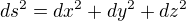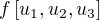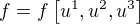## Calculating New Basis Vectors

Let x,y,z denote the cartesian coordinates of a point and,,its basis.

Given a transformation (from new coordinates to old) we want to find out the unit vectors (basis) of the new coordinate system.

Then the square of the length of a differential element ds is:Suppose we want to introduce a new coordinate system with orthogonal basis.

Let,,be the coordinates in this new coordinate system and,,be its basis vectors (which are to be calculated).

Then the same ds² is equal to:(From the above you can usually read off the values for,,).

(by virtue of the coordinate system being orthogonal, the mixed terms do not show up).

Hence, the differential path elementcan be written as:Which can be shown to hold by squaring.

Per total differential rule, the differential path elementis:[Equation I]

And so by comparing the coefficients:Or the more common way it is written:,,are called scale factors.

Let f be a scalar function of a position, i.e..

Then the Gradientis defined to be:Equivalently,This is the only valid definition of the gradient.

All other "gradients" need to be somehow defined on top of this one.

For example if you want to have a "u-gradient" which takes a functionand returns the gradient of the function, you have to connect it back to the original gradient.

Let's again make f a function of new coordinates.Then the total differential is:But these partial derivatives are NOT the gradient because the lengths would be wrong. The ONLY valid definition of the gradient is:(if it happens to be the partial derivative, fine. But it doesn't have to be).

By noting that the orthogonality of the basis vectors of the new coordinate system also holds in cartesian (!!!) coordinates, we get the following idea:

Let A, B, C be the components of the result of the gradient in the new coordinate system.Compare:Or how it is usually written:A,B,C are the components of the resulting vector in the new coordinate system.